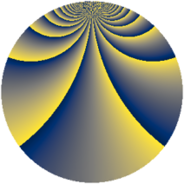# Properties

 Label 912.2.bnLevel $912$ Weight $2$ Character orbit 912.bn Rep. character $\chi_{912}(65,\cdot)$ Character field $\Q(\zeta_{6})$ Dimension $76$ Newform subspaces $15$ Sturm bound $320$ Trace bound $7$

# Related objects

## Defining parameters

 Level: $$N$$ $$=$$ $$912 = 2^{4} \cdot 3 \cdot 19$$ Weight: $$k$$ $$=$$ $$2$$ Character orbit: $$[\chi]$$ $$=$$ 912.bn (of order $$6$$ and degree $$2$$) Character conductor: $$\operatorname{cond}(\chi)$$ $$=$$ $$57$$ Character field: $$\Q(\zeta_{6})$$ Newform subspaces: $$15$$ Sturm bound: $$320$$ Trace bound: $$7$$ Distinguishing $$T_p$$: $$5$$, $$7$$, $$17$$

## Dimensions

The following table gives the dimensions of various subspaces of $$M_{2}(912, [\chi])$$.

Total New Old
Modular forms 344 84 260
Cusp forms 296 76 220
Eisenstein series 48 8 40

## Trace form

 $$76 q + 3 q^{3} + 4 q^{7} + q^{9} + O(q^{10})$$ $$76 q + 3 q^{3} + 4 q^{7} + q^{9} - 6 q^{13} + 3 q^{15} + 12 q^{19} - 12 q^{21} + 28 q^{25} + 10 q^{39} - 12 q^{43} - 14 q^{45} + 52 q^{49} + 3 q^{51} + 4 q^{55} - 17 q^{57} + 14 q^{61} + 26 q^{63} + 36 q^{67} - 18 q^{73} + 48 q^{79} - 19 q^{81} - 22 q^{85} - 30 q^{87} + 90 q^{91} + 2 q^{93} - 42 q^{97} + 8 q^{99} + O(q^{100})$$

## Decomposition of $$S_{2}^{\mathrm{new}}(912, [\chi])$$ into newform subspaces

Label Dim. $$A$$ Field CM Traces $q$-expansion
$$a_2$$ $$a_3$$ $$a_5$$ $$a_7$$
912.2.bn.a $$2$$ $$7.282$$ $$\Q(\sqrt{-3})$$ $$\Q(\sqrt{-3})$$ $$0$$ $$-3$$ $$0$$ $$-2$$ $$q+(-1-\zeta_{6})q^{3}-q^{7}+3\zeta_{6}q^{9}+(2+\cdots)q^{13}+\cdots$$
912.2.bn.b $$2$$ $$7.282$$ $$\Q(\sqrt{-3})$$ None $$0$$ $$0$$ $$6$$ $$4$$ $$q+(-1+2\zeta_{6})q^{3}+(2+2\zeta_{6})q^{5}+2q^{7}+\cdots$$
912.2.bn.c $$2$$ $$7.282$$ $$\Q(\sqrt{-3})$$ None $$0$$ $$3$$ $$-6$$ $$-2$$ $$q+(1+\zeta_{6})q^{3}+(-2-2\zeta_{6})q^{5}-q^{7}+\cdots$$
912.2.bn.d $$2$$ $$7.282$$ $$\Q(\sqrt{-3})$$ None $$0$$ $$3$$ $$-6$$ $$4$$ $$q+(2-\zeta_{6})q^{3}+(-2-2\zeta_{6})q^{5}+2q^{7}+\cdots$$
912.2.bn.e $$2$$ $$7.282$$ $$\Q(\sqrt{-3})$$ $$\Q(\sqrt{-3})$$ $$0$$ $$3$$ $$0$$ $$10$$ $$q+(1+\zeta_{6})q^{3}+5q^{7}+3\zeta_{6}q^{9}+(-6+\cdots)q^{13}+\cdots$$
912.2.bn.f $$2$$ $$7.282$$ $$\Q(\sqrt{-3})$$ None $$0$$ $$3$$ $$6$$ $$-2$$ $$q+(1+\zeta_{6})q^{3}+(2+2\zeta_{6})q^{5}-q^{7}+3\zeta_{6}q^{9}+\cdots$$
912.2.bn.g $$4$$ $$7.282$$ $$\Q(\sqrt{-2}, \sqrt{-3})$$ None $$0$$ $$-4$$ $$0$$ $$-8$$ $$q+(-1-\beta _{3})q^{3}+\beta _{1}q^{5}+(-2+2\beta _{1}+\cdots)q^{7}+\cdots$$
912.2.bn.h $$4$$ $$7.282$$ $$\Q(\sqrt{-2}, \sqrt{-3})$$ None $$0$$ $$-2$$ $$0$$ $$-8$$ $$q+(\beta _{1}-\beta _{2}-\beta _{3})q^{3}+\beta _{1}q^{5}+(-2+\cdots)q^{7}+\cdots$$
912.2.bn.i $$4$$ $$7.282$$ $$\Q(\sqrt{-3}, \sqrt{-11})$$ None $$0$$ $$-2$$ $$3$$ $$-2$$ $$q+(-1+\beta _{1}-\beta _{3})q^{3}+(1-\beta _{1})q^{5}+\cdots$$
912.2.bn.j $$4$$ $$7.282$$ $$\Q(\sqrt{-3}, \sqrt{-11})$$ None $$0$$ $$-1$$ $$-3$$ $$-2$$ $$q-\beta _{1}q^{3}+(-1-\beta _{3})q^{5}+(-\beta _{1}-\beta _{2}+\cdots)q^{7}+\cdots$$
912.2.bn.k $$4$$ $$7.282$$ $$\Q(\sqrt{-3}, \sqrt{-11})$$ None $$0$$ $$1$$ $$-9$$ $$-2$$ $$q+(\beta _{2}+\beta _{3})q^{3}+(-2+\beta _{1}-\beta _{2})q^{5}+\cdots$$
912.2.bn.l $$4$$ $$7.282$$ $$\Q(\sqrt{-3}, \sqrt{-11})$$ None $$0$$ $$2$$ $$9$$ $$-2$$ $$q+(\beta _{1}-\beta _{3})q^{3}+(2-\beta _{1}+\beta _{2})q^{5}+(-\beta _{1}+\cdots)q^{7}+\cdots$$
912.2.bn.m $$8$$ $$7.282$$ $$\Q(\zeta_{24})$$ None $$0$$ $$0$$ $$0$$ $$16$$ $$q+(\zeta_{24}-\zeta_{24}^{3}-\zeta_{24}^{4})q^{3}-\zeta_{24}^{5}q^{5}+\cdots$$
912.2.bn.n $$16$$ $$7.282$$ $$\mathbb{Q}[x]/(x^{16} - \cdots)$$ None $$0$$ $$-1$$ $$3$$ $$0$$ $$q-\beta _{4}q^{3}+(-\beta _{11}+\beta _{14})q^{5}+(-\beta _{5}+\cdots)q^{7}+\cdots$$
912.2.bn.o $$16$$ $$7.282$$ $$\mathbb{Q}[x]/(x^{16} - \cdots)$$ None $$0$$ $$1$$ $$-3$$ $$0$$ $$q+\beta _{7}q^{3}+(\beta _{11}-\beta _{14})q^{5}+(-\beta _{5}+\beta _{13}+\cdots)q^{7}+\cdots$$

## Decomposition of $$S_{2}^{\mathrm{old}}(912, [\chi])$$ into lower level spaces

$$S_{2}^{\mathrm{old}}(912, [\chi]) \cong$$ $$S_{2}^{\mathrm{new}}(57, [\chi])$$$$^{\oplus 5}$$$$\oplus$$$$S_{2}^{\mathrm{new}}(114, [\chi])$$$$^{\oplus 4}$$$$\oplus$$$$S_{2}^{\mathrm{new}}(228, [\chi])$$$$^{\oplus 3}$$$$\oplus$$$$S_{2}^{\mathrm{new}}(456, [\chi])$$$$^{\oplus 2}$$